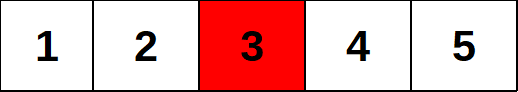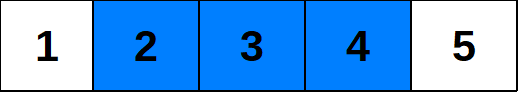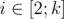# CF920A Water The Garden

• 60通过
• 139提交
• 题目来源
• 评测方式 RemoteJudge
• 标签 广度优先搜索,BFS 搜索 枚举,暴力
• 难度 入门难度
• 时空限制 1000ms / 256MB
• 提示：收藏到任务计划后，可在首页查看。

## 题意翻译

有一个长度为n的花园，一共有k个水龙头，分别在x，x...x[k]的位置。第t秒每个水龙头可以浇灌到它的前第t个方格和它后第t个方格，问浇灌完花园的时间。

感谢@伏轩彤666 提供的翻译

## 题目描述

It is winter now, and Max decided it's about time he watered the garden.

The garden can be represented as $n$ consecutive garden beds, numbered from $1$ to $n$ . $k$ beds contain water taps ( $i$ -th tap is located in the bed $x_{i}$ ), which, if turned on, start delivering water to neighbouring beds. If the tap on the bed $x_{i}$ is turned on, then after one second has passed, the bed $x_{i}$ will be watered; after two seconds have passed, the beds from the segment $[x_{i}-1,x_{i}+1]$ will be watered (if they exist); after $j$ seconds have passed ( $j$ is an integer number), the beds from the segment $[x_{i}-(j-1),x_{i}+(j-1)]$ will be watered (if they exist). Nothing changes during the seconds, so, for example, we can't say that the segment $[x_{i}-2.5,x_{i}+2.5]$ will be watered after $2.5$ seconds have passed; only the segment $[x_{i}-2,x_{i}+2]$ will be watered at that moment.The garden from test $1$ . White colour denotes a garden bed without a tap, red colour — a garden bed with a tap.The garden from test $1$ after $2$ seconds have passed after turning on the tap. White colour denotes an unwatered garden bed, blue colour — a watered bed. Max wants to turn on all the water taps at the same moment, and now he wonders, what is the minimum number of seconds that have to pass after he turns on some taps until the whole garden is watered. Help him to find the answer!

## 输入输出格式

输入格式：

The first line contains one integer $t$ — the number of test cases to solve ( $1<=t<=200$ ).

Then $t$ test cases follow. The first line of each test case contains two integers $n$ and $k$ ( $1<=n<=200$ , $1<=k<=n$ ) — the number of garden beds and water taps, respectively.

Next line contains $k$ integers $x_{i}$ ( $1<=x_{i}<=n$ ) — the location of $i$ -th water tap. It is guaranteed that for eachcondition $x_{i-1}<x_{i}$ holds.

It is guaranteed that the sum of $n$ over all test cases doesn't exceed $200$ .

Note that in hacks you have to set $t=1$ .

输出格式：

For each test case print one integer — the minimum number of seconds that have to pass after Max turns on some of the water taps, until the whole garden is watered.

## 输入输出样例

输入样例#1： 复制
3
5 1
3
3 3
1 2 3
4 1
1

输出样例#1： 复制
3
1
4


## 说明

The first example consists of $3$ tests:

1. There are $5$ garden beds, and a water tap in the bed $3$ . If we turn it on, then after $1$ second passes, only bed $3$ will be watered; after $2$ seconds pass, beds $[1,3]$ will be watered, and after $3$ seconds pass, everything will be watered.
2. There are $3$ garden beds, and there is a water tap in each one. If we turn all of them on, then everything will be watered after $1$ second passes.
3. There are $4$ garden beds, and only one tap in the bed $1$ . It will take $4$ seconds to water, for example, bed $4$ .
提示
标程仅供做题后或实在无思路时参考。
请自觉、自律地使用该功能并请对自己的学习负责。
如果发现恶意抄袭标程，将按照I类违反进行处理。